# Spatial Feature Engineering with ptools

#### 2022-12-27

This vignette goes over the different functions the ptools package has to generate spatial features common to criminological stype spatial covariate analysis. These include creating spatial units of analysis (grid cells, hexagons). And calculating different spatial feature engineering, such as the distance to nearest and kernel density estimates for covariate values.

## Creating Spatial Units of Analysis

I will start off with an example loading in the data that is included in the package, and I load the sp library to use its simple plotting functions.

library(ptools)
library(sp)     #for plotting functions
library(raster) #for buffer later on

# Plot shootings in NYC
plot(nyc_bor)
plot(nyc_shoot,pch='.',add=TRUE)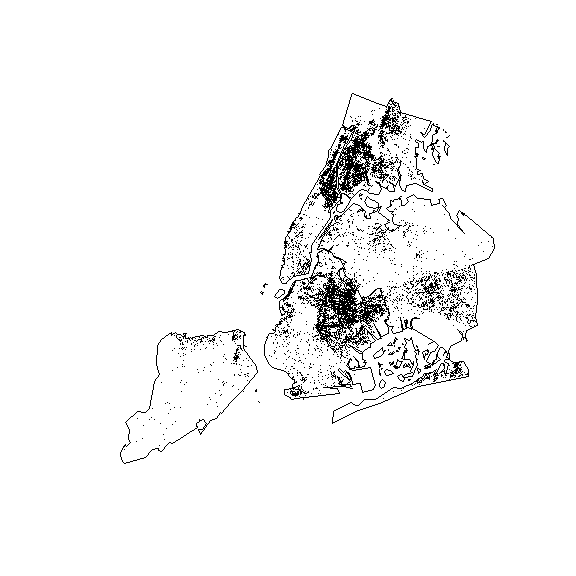We can create a set of vector grid cells over the study area. The projection is in feet, so here I make half-square mile grid cells over the NYC borough outline.

hm_grid <- prep_grid(nyc_bor,5280/2)

# Plot shootings in NYC
plot(nyc_bor)
plot(hm_grid,col='grey',border='white',add=TRUE)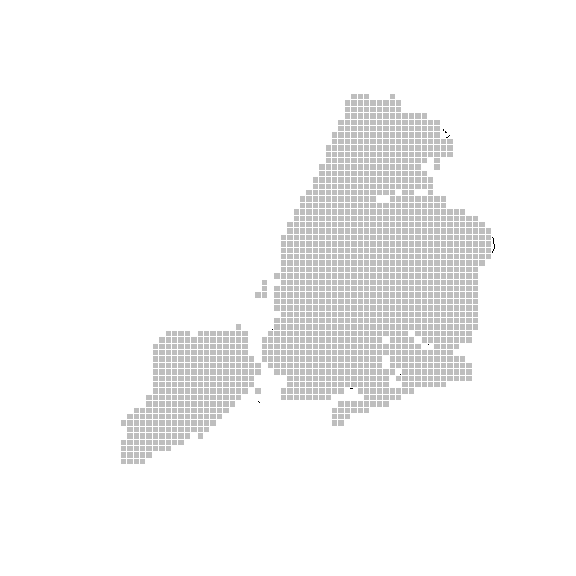I have provided two arguments to the functions to limit the vector areas returned. One is sometimes we only want areas that have at least one observation for certain analyses (so at least one shooting over the 15+ year period in the current sample).

lim_grid <- prep_grid(nyc_bor,5280/2,point_over=nyc_shoot)

# Plot shootings in NYC
plot(nyc_bor)
plot(lim_grid,col='grey',border='white',add=TRUE)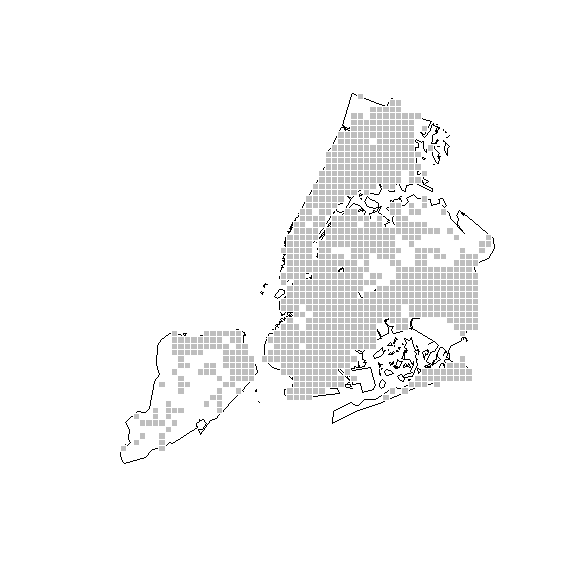So you can see it is not as filled in for Staten Island. Note that there is an additional argument, point_n, if you wanted to limit grid cells even more, so at least 2, 3, etc. points are in a grid cell.

A second way we can limit the grid cells are to not have dongle grid cells – cells that only cover a small portion of the city. Here I only include grid cells that have over 30% of their area inside of the border of the city. Note though that this will cause some areas inside the jurisdiction to not be covered. But you can pretty clearly see the reduced number of areas compared to the original grid cells.

lim_grid2 <- prep_grid(nyc_bor,5280/2,clip_level = 0.3)

# Plot shootings in NYC
plot(nyc_bor)
plot(lim_grid2,col='grey',border='white',add=TRUE)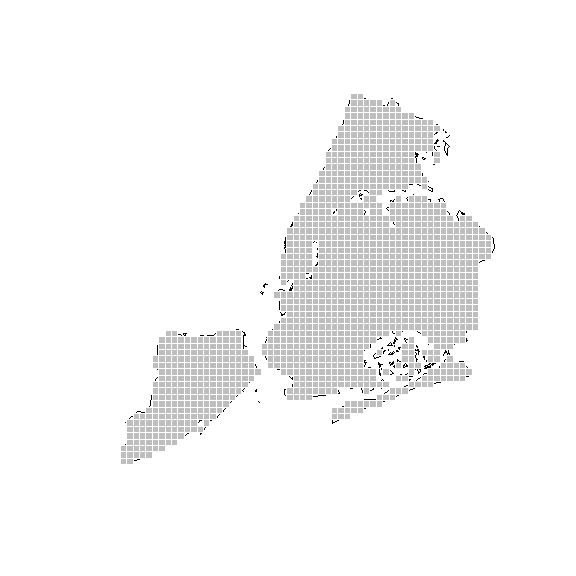# See how many fewer than original
print(nrow(hm_grid))   #original
#>  1493
print(nrow(lim_grid2)) #no dongles less than 30%
#>  1290
print(nrow(lim_grid))  #only overlap 1 shooting
#>  867

I also have a similar function, prep_hexgrid, that prepares spatial units of analysis in hexagons instead of square grid cells. One difference is that instead of specifying the size of the cell per length of a side, it specifies it per unit area. So it make it a square mile, use 5280^2 as the area argument. (Here I make it a half square mile.)

hex_grid <- prep_hexgrid(nyc_bor,(5280/2)^2,clip_level = 0.3)
#> Warning in proj4string(obj): CRS object has comment, which is lost in output; in tests, see
#> https://cran.r-project.org/web/packages/sp/vignettes/CRS_warnings.html

# Plot shootings in NYC
plot(hex_grid,col='lightblue',border='white')
plot(nyc_bor,lwd=2,add=TRUE)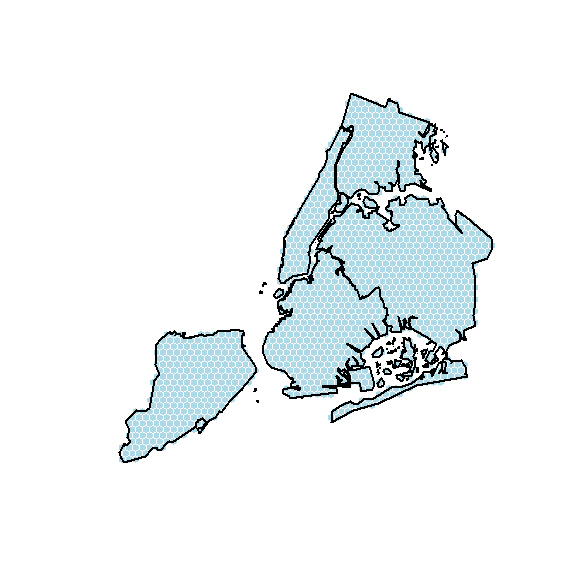The prep_hexgrid function also has the same point_over and point_n arguments as does the prep_grid function. Here I limit to areas with more than 4 shootings.

hex_lim <- prep_hexgrid(nyc_bor,(5280/2)^2,point_over=nyc_shoot,point_n=4)
#> Warning in proj4string(obj): CRS object has comment, which is lost in output; in tests, see
#> https://cran.r-project.org/web/packages/sp/vignettes/CRS_warnings.html

# Plot shootings in NYC
plot(hex_lim,col='lightblue',border='white')
plot(nyc_bor,lwd=2,add=TRUE)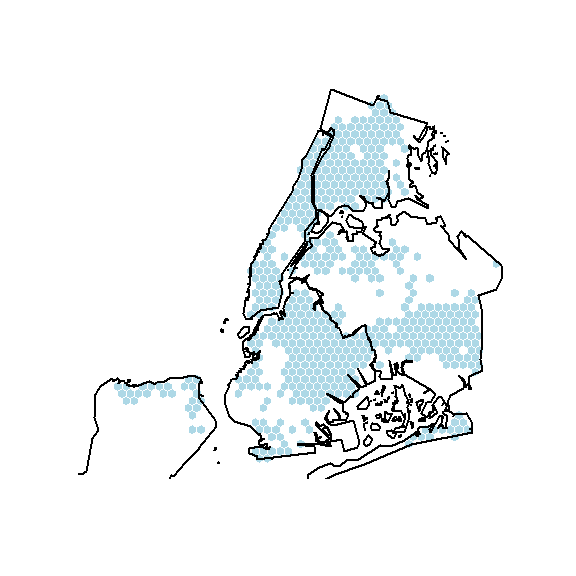Note that the way I wrote these functions, the clip_level for hexagons can be somewhat slow. Doing point_over though should be reasonably fast. prep_grid converts from raster to vector, so if you have very tiny cells, you may consider just keeping the original raster format for analysis. The conversion to vector will always creep up your memory usage and computation time.

Finally, I have a function that if you have a set of origin points, you can create voronoi polygons within an outline area. Sometimes if working with line or address based features it is simpler to convert to polygons and do subsequent geospatial operations. But note this can take a bit of time as well for any really dense point set (same as really tiny grid cells or hexagons).

# Make a smaller sample of the liquor stores in NYC
liq_samp <- nyc_liq[sample(rownames(nyc_liq@data),20),]
# Buffer, it is hard to view all the little islands
nyc_buff <- buffer(nyc_bor,5000)
# Now create Voronoi/Thiessen areas
liq_vor <- vor_sp(nyc_buff,liq_samp)
#> Warning in sp::proj4string(outline): CRS object has comment, which is lost in output; in tests, see
#> https://cran.r-project.org/web/packages/sp/vignettes/CRS_warnings.html

# Plot to show off
plot(liq_vor,col='tan',border='white')
plot(nyc_buff,border='black',lwd=2,add=TRUE)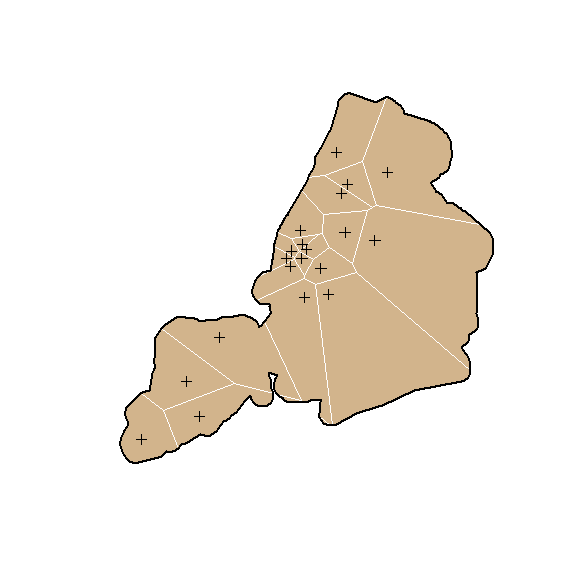## Spatial Feature Engineering

The spatial feature engineering functions are not only for creating vector spatial units, but assigning typical values we may be interested in. So lets reuse our hex_grid over the city, and calculate the number of shootings in each hexgrid:

hex_grid$shoot_cnt <- count_xy(hex_grid,nyc_shoot) spplot(hex_grid,zcol='shoot_cnt')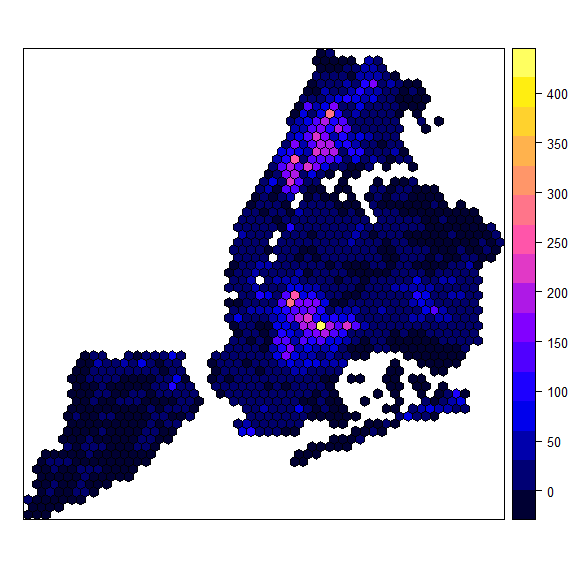Remember since hex_grid eliminated some dongles, we may be interested to see if we happened to lose some shootings on the edges of NYC. print(sum(hex_grid$shoot_cnt))
#>  26935
print(nrow(nyc_shoot))
#>  26996

So we ended up losing 37 shootings using this grid. We can also use weights in these functions. So here, say I wanted to do a pre/post analysis. A simple way to do that is to weight the observations in the original point pattern, and then use that field as a weight.

covid_date <- as.Date('03/22/2020', format="%m/%d/%Y")
nyc_shoot$pre <- 1*(nyc_shoot$OCCUR_DATE < covid_date)
nyc_shoot$post <- 1*(nyc_shoot$OCCUR_DATE >= covid_date)
hex_grid$shoot_pre <- count_xy(hex_grid,nyc_shoot,weight='pre') hex_grid$shoot_post <- count_xy(hex_grid,nyc_shoot,weight='post')
plot(hex_grid$shoot_pre,hex_grid$shoot_post)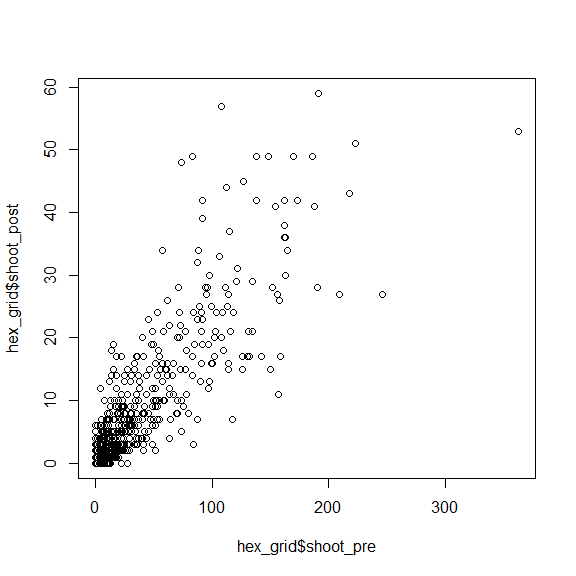So you can see that the pre and post shooting counts are highly correlated and a few maybe outliers.

Sometimes we want other feature engineering RTM or Egohood style, with density of nearby features. So we can calculate the density (assuming a Gaussian kernel), for liquor stores at the centroid of our polygon features. Since the area of our hexgrid is half a square mile, (5280/2)^2, this means that the vertex to vertex diameter of our hexagon cells is hex_dim((5280/2)^2), not quite 3300 feet (see also the hex_area and hex_wd functions to convert between different hexagon sizes). So there is not much point of doing a KDE bandwidth for under this (will basically be the same as just counting up the total inside of the hexagon. So here I do a bandwidth of 1 mile.

hex_grid$kliq_1mile <- kern_xy(hex_grid,nyc_liq,5280) spplot(hex_grid,zcol='kliq_1mile')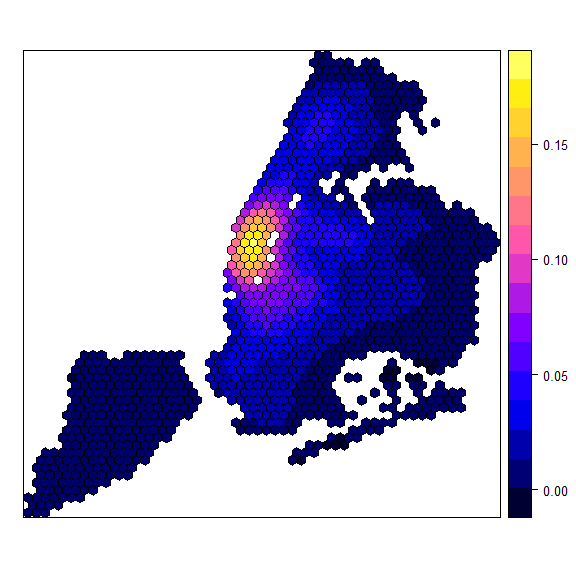I also have similar functions to kernel density for bisquare weights, bisq_xy, and for inverse distance weighting, idw_xy. And for these you can pass in weights the same as for the counts I showed above. I don’t have any demographic data here, but for egohoods you may have census data at centroids, such as the total number households in poverty, and use that as a weight (or a mean estimate). One function that is slightly different than others is the distance between two point sets. So here is the distance to the nearest NYC outdoor cafe (note that this data source has no outdoor cafes in Staten Island): hex_grid$dist_cafe <- dist_xy(hex_grid,nyc_cafe)
spplot(hex_grid,zcol='dist_cafe')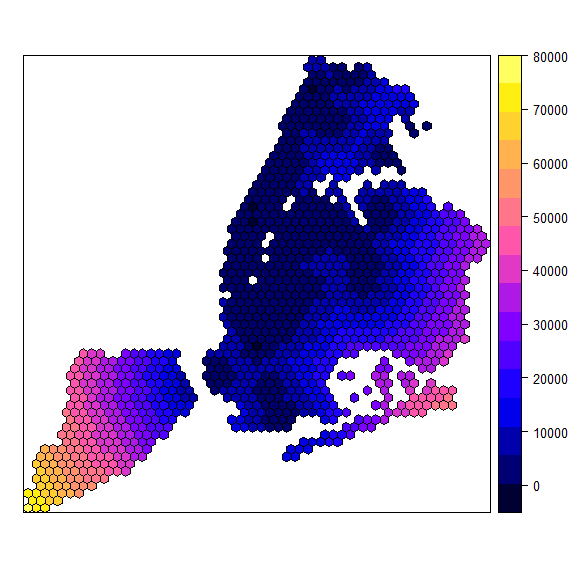Unlike the other functions, you can pass in two point sets here, under the hood it just grabs the X/Y coordinates for each set. So here is the closest shooting to a cafe.

dist_shoot <- dist_xy(nyc_cafe,nyc_shoot,fxy=c('X_COORD_CD','Y_COORD_CD'))
summary(dist_shoot)
#>    Min. 1st Qu.  Median    Mean 3rd Qu.    Max.
#>   33.23  272.49  495.54  597.37  835.83 5765.32

Not shown, but if the base polygons/features do not have X/Y coordinates. You can do something like coords <- coordinates(base); base$x <- coords[,1] and ditto the second column for y (assuming base is a sp class object). This function does not have a weight function, as that does not make sense. The final feature engineering point to note is the dcount_xy function. Unlike the other functions that operate on the centroids of the polygons (or whatever X/Y coordinates you pass in), this counts the number of points within a specified distance to the polygon border. This buffers the feature dataset and then calculates the overlap, so with very large features can take a bit (whereas kernel density calc timing is mostly a function of how many base polygons you have): hex_grid$dcnt_cafe1mile <- dcount_xy(hex_grid,nyc_cafe,5280)
#> Warning in RGEOSBinPredFunc(spgeom1, spgeom2, byid, func): spgeom1 and spgeom2
#> have different proj4 strings
plot(hex_grid$dist_cafe,hex_grid$dcnt_cafe1mile,xlim=c(0,10000))
abline(v=5280) # not perfect, because of difference between polygon distance vs centroid distance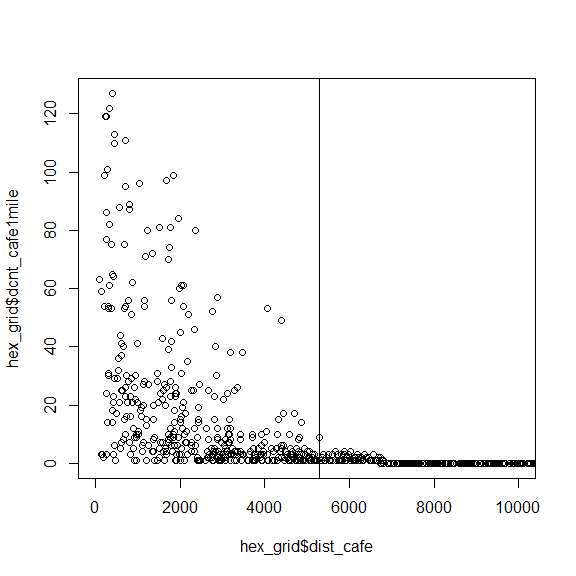Again you can use a weight function in the dcount_xy function. So if you wanted to say count the number of children attending school in 1 kilometer of your base polygons, this would be the function you use.